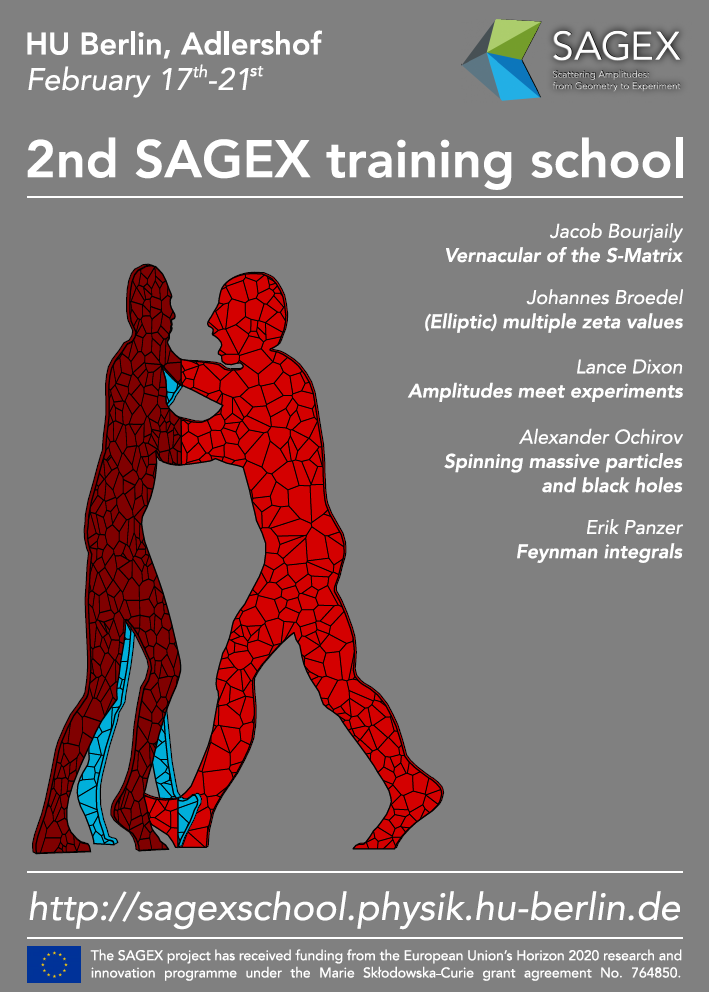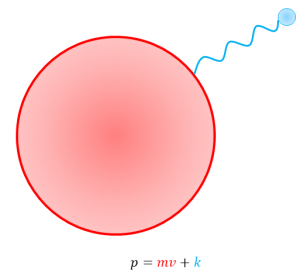## We are hiring!

SAGEX is recruiting for an EU Project Manager (Maternity Cover). The role is based at Queen Mary University of London (Mile End Road Campus) and is fixed-term for 11 months, with a start date of mid-October. Find out how to apply.

## Register for the 2nd SAGEX training school

The second SAGEX training school will take place from  17-21 February 2020, at Humboldt University. It is open to external students as well as our SAGEX ESRs. Advance registration is required.## On the Polygon Front Lines: Visualising the Amplituhedron with the Wolfram Language

SAGEX Early Stage Researcher Gabriele Dian recently spent three months on secondment at Wolfram Research Inc. During his secondment he worked on visualisations of the amplituhedron using Mathematica and wrote a post about his work for the Wolfram blog. Read Gabriele’s blog post.

## Classical Gravitation from Quantum Scattering Amplitudes

Based on the article ‘Heavy Black Hole Effective Theory‘, P. H. Damgaard,

Over a century after its formulation, predictions of Einstein’s theory of General Relativity, describing gravitational interactions, continue to be verified experimentally. One of the predicted phenomena is gravitational waves (GWs), gravitational radiation emitted when gravitationally interacting bodies orbit one another, and whose propagation in space results in the contraction and expansion of space itself. Their observation for the first time in 2015 by the LIGO and VIRGO collaborations, from the collision of two black holes, has attracted much renewed attention from the scattering amplitudes community.

General Relativity is a theory of classical physics; that is to say it describes processes that take place on large distance scales, where quantum mechanical effects are negligible. With this in mind, it is natural to wonder what a classical phenomenon such as the emission of GWs has to do with scattering amplitudes, which describe the interactions of quantum mechanical particles. The conventional wisdom in quantum field theory is that only the terms in a scattering amplitude that contain the fewest powers of a theory’s coupling constant (the so-called tree-level amplitudes) encode classical effects. While it is true that tree-level amplitudes contain classical effects – the classical Newtonian gravitational force and the Coulomb electromagnetic force can both derived from tree-level amplitudes – it was shown in the mid 90s that terms with higher powers of the coupling constant (sub-leading, or loop-level amplitudes) can also contain classical corrections to long-distance physics! In particular, one can use scattering amplitudes to correct the interaction potential between two bodies subject to, say, Newton’s law of gravitation.

This hints at a connection between the amplitudes community and those interested in classical gravitational phenomena such as GWs. By calculating gravitational amplitudes that are more and more sub-leading, one can extract ever higher order corrections to the classical gravitational interaction potential between two bodies. With recent advances in the computational efficiency of scattering amplitudes, it can even be easier to obtain these corrections using quantum field theory than using Einstein’s General Relativity! Furthermore, there is a sense in which it is appropriate to identify quantum mechanical particles with black holes: similarly to these particles, black holes are entirely describable in terms of their mass and how fast they spin around themselves. With the interpretation of scattering amplitudes describing the interactions of black holes, the corrections to the gravitational interaction potential can be processed to improve the theoretical predictions of what signal a gravitational wave coming from colliding black holes should produce in experiments.

While the scattering amplitudes approach appears to have much applicability to classical physics, the computation of classical effects from scattering amplitudes remains an inefficient task. When an amplitude is computed, the vast majority of terms are only relevant to quantum mechanical systems. Someone who is interested in classical physics then needs to identify and isolate which terms in the amplitude represent the sought after classical corrections. There are reliable techniques for doing this, but it is natural to wonder if one can avoid having to compute the quantum portion of the amplitude in the first place. Heavy Black Hole Effective Theory (HBET) was formulated with this question in mind.Figure 1: A bound state of one heavy quark (red) and one light quark (blue), with interactions mediated via the strong force (wavy line). The total momentum p is decomposed into a large part mv, where m is the mass of the heavy quark and v is its velocity, and a small residual momentum |k| << m that parameterises the energy and momentum carried by the light quark and by the particles mediating the strong force.

HBET is inspired by a well-established effective quantum field theory called Heavy Quark Effective Theory (HQET). HQET was formulated to describe systems where a heavy quark is in a bound state with a much lighter quark, which interact via the strong force. The characteristic property of HQET is that the total momentum of the system is decomposed into a large part describing the energy and momentum of the heavy quark, and a small residual momentum which contains the energy and momentum of the light quark and the energy due to the interactions between the light and heavy quark (see Figure 1). The residual momentum is the key to connecting this momentum decomposition with the question at hand: it can be argued that the “classicality” of terms in the amplitudes depends on how many factors of the residual momentum they carry. When deriving the building blocks of a scattering amplitude – the so-called Feynman rules – from HQET, one can then keep only terms that contain the correct number of factors of the residual momentum to contribute to classical terms in the amplitude.

These same properties of HQET can be applied to gravitationally interacting systems, resulting in HBET. In the linked article, the residual momentum was reinterpreted to represent the momentum transfer between two bodies (see Figure 2), and the understanding of the residual momentum as parameterising classicality was applied to the gravitational scattering of two spin-1/2 particles. It was shown there that known classical results (and some new ones) could be obtained by using only the portions of the Feynman rules with a predetermined number of residual momentum factors. Crucially, there was no need to compute entire scattering amplitudes, or to manually separate classical from quantum terms in the amplitude at any point in the calculation.Figure 2: A system of two interacting black holes, with interactions mediated via the gravitational force (wavy line). The total momentum of each black hole p1 and p2 is decomposed into a large part m|v|, where mi is the mass of black hole i and vi is its velocity, and a small residual momentum |k| << mi that parameterises the energy and momentum transferred in the gravitational interaction. The residual momentum flows from the black hole on the left to the one on the right.

The developments of HBET have answered the question we set out to understand: it is indeed unnecessary to compute an entire quantum scattering amplitude when one is only interested in classical physics. Nevertheless, our work is not done. HBET has so far only been formulated for spin-0 and 1/2 particles. If we are interested in describing classical black holes, it is essential to be able to describe a system with arbitrary values of spin. There are two ways to achieve this: the first is to explicitly formulate models for every spin that allow us to extract the desired Feynman rules for a given spin – an approach which is quite cumbersome. Alternatively, modern approaches to scattering amplitudes allow one to construct amplitudes using minimal building block amplitudes that can be easily generalized to arbitrary spin. Expressing the degrees of freedom of HBET in terms of these minimal amplitudes would provide a much more tractable and elegant extension to any spin. Whichever direction future studies take, one thing is certain: quantum scattering amplitudes have much to say about classical physics.

Cover image courtesy of the LIGO collaboration.